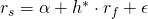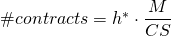# Optimal hedge ratio

Companies and the portfolio of investors are exposed to various amounts of risk factors. They can opt to reduce their exposure to a certain risk factor. For example oil, gold or other commodity prices or foreign exchange risk. Of course, anyone wants to reduce their volatility as much as possible at the most favorable cost and without over-or underhedging themselves. In order to achieve this, it is needed to calculate what is called the optimal hedge ratio. In what follows we explain the basics based on the optimal hedge ratio how  a portfolio can be hedged against a certain risk factor by using futures.

## Optimal hedge ratio calculation

The optimal hedge ratio, h*, quantifies the systematic comovement between 2 assets. It is called optimal since a position in future contracts of h* minimizes the portfolio variance most. It can be calculated based on the standard deviation of both the spot and future price and their correlation.Alternatively, it can be obtained through regression the spot returns on the future returns.## Amount of contracts

After having determined the optimal hedge ratio, you need to calculate the amount of contracts in order to hedge a certain amount. The amount of future contracts you need to obtain equals the hedging amount, M, divided by the contract size of the future, CS, multiplied by the optimal hedge ratio.## Summary

Hedging can reduce the risk exposure of a portfolio to a certain risk factor. The optimal hedge ratio determines how strong the relationship is between 2 assets which can be exploited to achieve the minimum portfolio variance. Based on the contract amount of futures, the optimal hedge ratio determines the amount of futures that should be bought or sold.